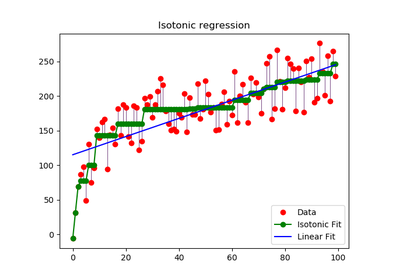# `sklearn.isotonic`.IsotonicRegression¶

class `sklearn.isotonic.``IsotonicRegression`(y_min=None, y_max=None, increasing=True, out_of_bounds=’nan’)[source]

Isotonic regression model.

The isotonic regression optimization problem is defined by:

```min sum w_i (y[i] - y_[i]) ** 2

subject to y_[i] <= y_[j] whenever X[i] <= X[j]
and min(y_) = y_min, max(y_) = y_max
```
where:
• `y[i]` are inputs (real numbers)
• `y_[i]` are fitted
• `X` specifies the order. If `X` is non-decreasing then `y_` is non-decreasing.
• `w[i]` are optional strictly positive weights (default to 1.0)

Read more in the User Guide.

Parameters: y_min : optional, default: None If not None, set the lowest value of the fit to y_min. y_max : optional, default: None If not None, set the highest value of the fit to y_max. increasing : boolean or string, optional, default: True If boolean, whether or not to fit the isotonic regression with y increasing or decreasing. The string value “auto” determines whether y should increase or decrease based on the Spearman correlation estimate’s sign. out_of_bounds : string, optional, default: “nan” The `out_of_bounds` parameter handles how x-values outside of the training domain are handled. When set to “nan”, predicted y-values will be NaN. When set to “clip”, predicted y-values will be set to the value corresponding to the nearest train interval endpoint. When set to “raise”, allow `interp1d` to throw ValueError. X_min_ : float Minimum value of input array X_ for left bound. X_max_ : float Maximum value of input array X_ for right bound. f_ : function The stepwise interpolating function that covers the domain X_.

Notes

Ties are broken using the secondary method from Leeuw, 1977.

References

Isotonic Median Regression: A Linear Programming Approach Nilotpal Chakravarti Mathematics of Operations Research Vol. 14, No. 2 (May, 1989), pp. 303-308

Isotone Optimization in R : Pool-Adjacent-Violators Algorithm (PAVA) and Active Set Methods Leeuw, Hornik, Mair Journal of Statistical Software 2009

Correctness of Kruskal’s algorithms for monotone regression with ties Leeuw, Psychometrica, 1977

Methods

 `fit`(X, y[, sample_weight]) Fit the model using X, y as training data. `fit_transform`(X[, y]) Fit to data, then transform it. `get_params`([deep]) Get parameters for this estimator. `predict`(T) Predict new data by linear interpolation. `score`(X, y[, sample_weight]) Returns the coefficient of determination R^2 of the prediction. `set_params`(**params) Set the parameters of this estimator. `transform`(T) Transform new data by linear interpolation
`__init__`(y_min=None, y_max=None, increasing=True, out_of_bounds=’nan’)[source]
`X_`

DEPRECATED: Attribute `X_` is deprecated in version 0.18 and will be removed in version 0.20.

`fit`(X, y, sample_weight=None)[source]

Fit the model using X, y as training data.

Parameters: X : array-like, shape=(n_samples,) Training data. y : array-like, shape=(n_samples,) Training target. sample_weight : array-like, shape=(n_samples,), optional, default: None Weights. If set to None, all weights will be set to 1 (equal weights). self : object Returns an instance of self.

Notes

X is stored for future use, as transform needs X to interpolate new input data.

`fit_transform`(X, y=None, **fit_params)[source]

Fit to data, then transform it.

Fits transformer to X and y with optional parameters fit_params and returns a transformed version of X.

Parameters: X : numpy array of shape [n_samples, n_features] Training set. y : numpy array of shape [n_samples] Target values. X_new : numpy array of shape [n_samples, n_features_new] Transformed array.
`get_params`(deep=True)[source]

Get parameters for this estimator.

Parameters: deep : boolean, optional If True, will return the parameters for this estimator and contained subobjects that are estimators. params : mapping of string to any Parameter names mapped to their values.
`predict`(T)[source]

Predict new data by linear interpolation.

Parameters: T : array-like, shape=(n_samples,) Data to transform. T_ : array, shape=(n_samples,) Transformed data.
`score`(X, y, sample_weight=None)[source]

Returns the coefficient of determination R^2 of the prediction.

The coefficient R^2 is defined as (1 - u/v), where u is the residual sum of squares ((y_true - y_pred) ** 2).sum() and v is the total sum of squares ((y_true - y_true.mean()) ** 2).sum(). The best possible score is 1.0 and it can be negative (because the model can be arbitrarily worse). A constant model that always predicts the expected value of y, disregarding the input features, would get a R^2 score of 0.0.

Parameters: X : array-like, shape = (n_samples, n_features) Test samples. y : array-like, shape = (n_samples) or (n_samples, n_outputs) True values for X. sample_weight : array-like, shape = [n_samples], optional Sample weights. score : float R^2 of self.predict(X) wrt. y.
`set_params`(**params)[source]

Set the parameters of this estimator.

The method works on simple estimators as well as on nested objects (such as pipelines). The latter have parameters of the form `<component>__<parameter>` so that it’s possible to update each component of a nested object.

Returns: self :
`transform`(T)[source]

Transform new data by linear interpolation

Parameters: T : array-like, shape=(n_samples,) Data to transform. T_ : array, shape=(n_samples,) The transformed data
`y_`

DEPRECATED: Attribute `y_` is deprecated in version 0.18 and will be removed in version 0.20.

## Examples using `sklearn.isotonic.IsotonicRegression`¶Isotonic Regression